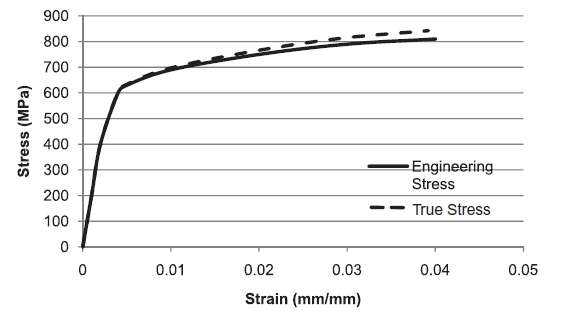### Stress: Other Measures of Stress

### Engineering vs True Stress:

During material uniaxial tests, the value of the applied stress is obtained by dividing the applied force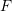by the measured initial cross sectional area of the specimen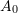. In this case, the stress is termed the “Engineering Stress”. If excessive decrease (or increase) in the cross sectional area occurs, then the force should be divided by the actual area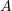(area after deformation) to obtain the “True Stress”: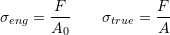Similarly, the “Engineering Strain” as defined in uniaxial strain measures is obtained by dividing the elongation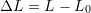by the original length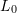, while the “True Strain” is obtained by taking the natural logarithm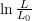: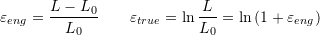Considering the behaviour of metals, at higher strains, the deformation of a metal is volume preserving and thus: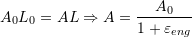In this case, the true stress is related to the engineering stress as follows: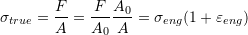#### Example:

The engineering stress vs. the engineering strain of a metal is given by the following table:

 Engineering Strain (%) 0 0.1 0.2 0.4 0.5 1 2 3 4 Engineering Stress(MPa) 0 200 400 600 630 690 750 790 810

Compute the true stress and the true strain. Plot the engineering and the true stress vs. strain relationships.

##### Solution:

The following table is constructed using the equations for the true stress and true strain: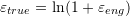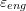(mm/mm) 0 0.001 0.002 0.004 0.005 0.01 0.02 0.03 0.04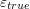0 0.001 0.001998 0.00399 0.004988 0.00995 0.0198 0.02956 0.0392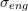(MPa) 0 200 400 600 630 690 750 790 810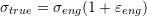(MPa) 0 200.2 400.8 602.4 633.15 696.9 765 813.7 842.4

As shown in the plot below, the values of the true stress for metals are usually slightly higher than the values of the engineering stress for strains higher than 0.005.